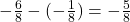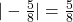## (01.02MC)Point A is located at negative 6 over 8 and point B is located at negative 1 over 8. What is the distance between points A and B?

Question

(01.02MC)Point A is located at negative 6 over 8 and point B is located at negative 1 over 8. What is the distance between points A and B?

negative 6 over 8 plus negative 1 over 8 = negative 7 over 8; therefore, the distance from A to B is absolute value of negative 7 over 8 equals negative 7 over 8 units
negative 6 over 8 plus negative 1 over 8 = negative 7 over 8; therefore, the distance from A to B is absolute value of negative 7 over 8 equals 7 over 8 units
negative 6 over 8 minus negative 1 over 8 = negative 5 over 8; therefore, the distance from A to B is absolute value of negative 5 over 8 equals negative 5 over 8 units
negative 6 over 8 minus negative 1 over 8 = negative 5 over 8; therefore, the distance from A to B is absolute value of negative 5 over 8 equals 5 over 8 units

in progress 0
6 months 2021-08-09T14:54:48+00:00 1 Answers 10 views 0